Next: 7.2 Stress Calculation Concept Up: 7. Stress Dependent Oxidation Previous: 7. Stress Dependent Oxidation

# 7.1 Oxidation Modeling with Stress

There are two parameters in the oxidation model, which are influenced by stress. The first one is the stress dependent diffusion coefficient [109,110]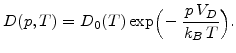(7.1)

Here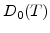is the low stress diffusion coefficient (3.9),is the pressure in the respective material,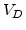is the activation volume,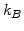is the Boltzmann's constant, and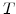is the temperature in Kelvin.
The second parameter is the stress dependent strength of a spatial sink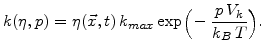(7.2)

Both parameters are exponentially reduced with pressure, which is only valid for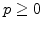.

With these two stress dependent parameters the three main equations in the oxidation model, which describe the oxidant diffusion (3.2), the-dynamics (3.5), and the volume increase (3.8), become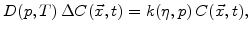(7.3)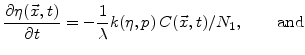(7.4)(7.5)

The stress is generally described with the formula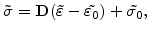(7.6)

where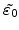stands for the desired volume increase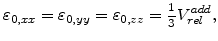(7.7)

and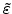represents the actual volume expansion, because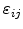are the partial derivatives of the actual displacements (3.21). On a finite element the mechanical problem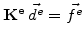is loaded by the desired volume increase (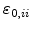-values) which leads to the internal forces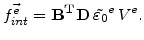(7.8)

The actual displacements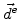are obtained after solving the mechanical system (see Fig. 5.5). With these results the actual strains can be calculated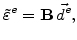(7.9)

and the stress on an element can be determined with (7.7).

A worth mentioning aspect is the visco-elastic stress computation in the FEDOS simulation procedure. For the actual time step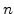the visco-elastic stress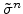is the sum of a dilatation and a deviatoric part, because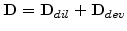as depicted in Section 3.2.5.2. Therefore, also the residual stress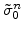for the actual time stepconsists of a dilatation and a deviatoric part so that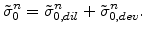(7.10)

The components of the actual residual stress tensor are build up from the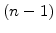previous time steps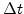according to (7.12) for the dilatation and (7.13) for the deviatoric part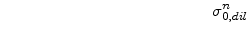(7.11)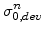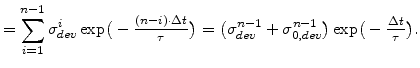(7.12)

An important characteristic of visco-elastic materials is the stress relaxation of the deviatoric stress components over time with the Maxwellian relaxation time constant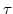, as given in (7.13). The recursive form for residual stress calculation in the right hand side of (7.12) and (7.13) offers the benefit that the residual stress parts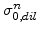and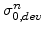at actual time stepcan be simply computed by adding the components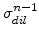and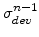from the last stepto the already existing residual stress parts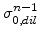and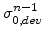determined at previous step.

In contrast to stress the pressure needed for (7.1) and (7.2) is a scalar. It is positive, if the com-pressive stress components which have a negative sign, are predominant. So pressure always has an opposite sign compared to stress. The pressure is the average of the stress tensors trace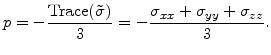(7.13)Next: 7.2 Stress Calculation Concept Up: 7. Stress Dependent Oxidation Previous: 7. Stress Dependent Oxidation

Ch. Hollauer: Modeling of Thermal Oxidation and Stress Effects